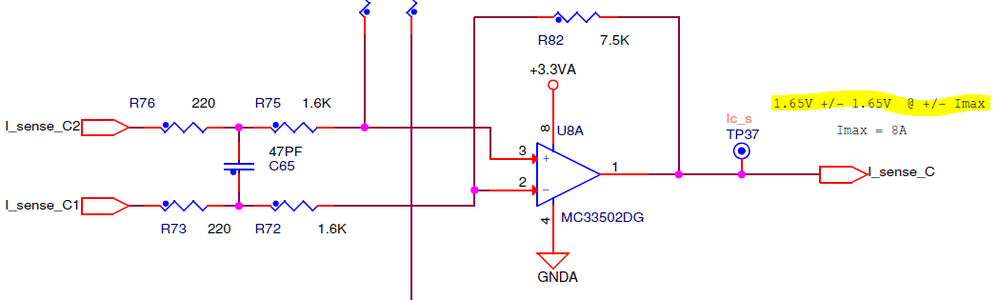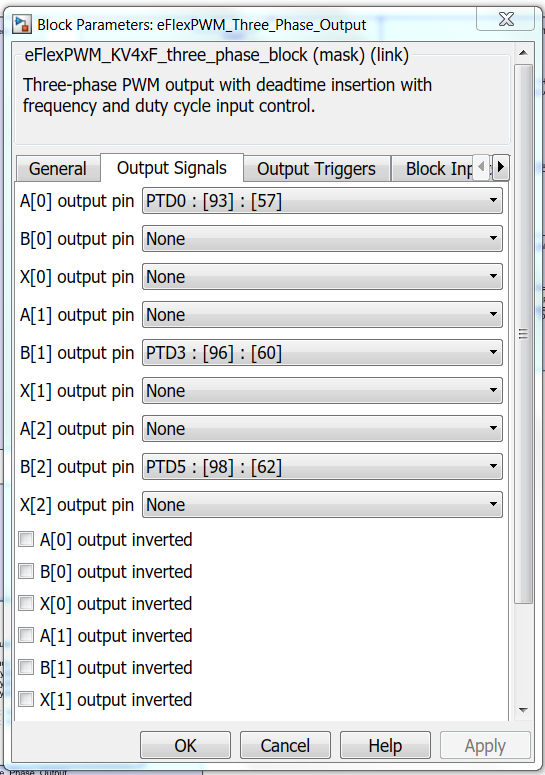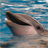# current measurements with HVP-MC3PH

cancel
Showing results for
Did you mean:

## current measurements with HVP-MC3PH

827 ViewsContributor V

Hi,

Like it is given in the circuit diagram I excpect a ADC value about 2048 with no load (1,65V Offset).

Now I've connected a resistor between Phase A and C Pins.The PWM_Three_Phase_Output Signals are configured like this:If I switch on the PWM I obtain a negative current in Phase C (ADC value < 2048), but I think it has to be positive.

Is there an error in the documentaion, so it has to be inverted ?

4 Replies
490 ViewsNXP TechSupport

Hi, Leon,

First of all, let's derivate the AMP output voltage. Assume that the I_SENSE_C2 voltage is VC2, the  I_SENSE_C1 voltage is VC1, the pin2 and pin3 voltage of AMP is  V23, the AMP output voltage(pin1 of AMP) is V1. Because the pin3 of AMP is connected to a 1.65V  voltage reference, based on ZERO theory, we can get the following equation:

(1.65-V23)/R83=(V23-VC2)/(R76+R75)

(V1-V23)/R82=(V23-VC1)/(R73+R72)

From the above two equations, we can get

V1/R82=1.65/R83+VC2/(R76+R75)-VC1/(R73+R72)

After we replacing the R value

V1=1.65+(7.5/1.82)*(VC2-VC1)=1.65+4.12*(VC2-VC1).

Because the ADC can only sample positive voltage, so the V1 must be greater than zero.  In other words, the (VC2-VC1) must be greater than -0.4V and less than +0.4V.

Each ADC channel has OFFESET register, you can set the ADC OFFSET register as 0x4000, which represents 1.65V, so the ADC will get the actual voltage.

BR

Xiangjun rong

490 ViewsContributor V

I still need to know the answer for my question.

490 ViewsNXP Employee

Hi lethuer2‌,

What you read from the ADC is normal. In SW you need to do the inversion of the current if you wish to keep the generic motor control conventions. A current flowing into the motor winding has a positive polarity while the current flowing out of the motor winding is negative.

On the shunt - a positive voltage drop means the current flows out of the motor winding (since the shunt is mounted on the lower inverter legs).

Therefore, you need to invert the value of the ADC readings in SW.

Hope it helps!

Daniel

490 ViewsContributor V

Okay, so the hint in the circuit diagram should be like this ?

1.65V +/- @ -/+ Imax